# Newton Forward And Backward Interpolation

Interpolation is the technique of estimating the value of a function for any intermediate value of the independent variable, while the process of computing the value of the function outside the given range is called extrapolation.

Forward Differences : The differences y1 – y0, y2 – y1, y3 – y2, ……, yn – yn–1 when denoted by dy0, dy1, dy2, ……, dyn–1 are respectively, called the first forward differences. Thus the first forward differences are :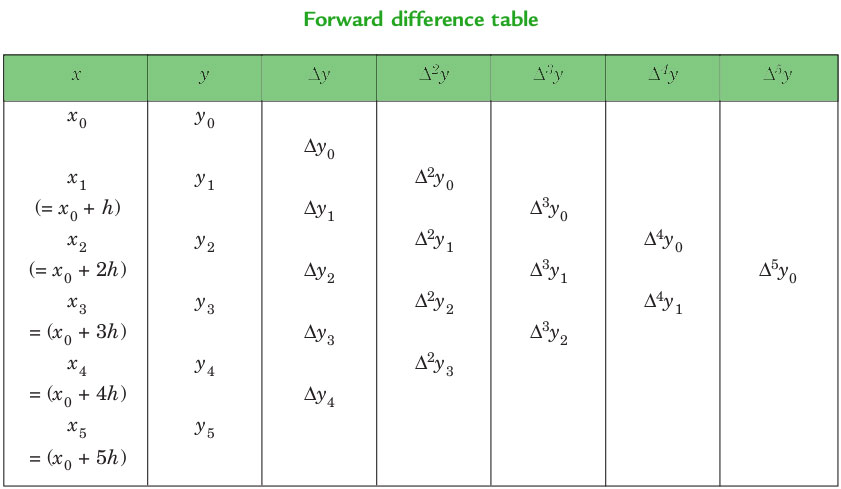NEWTON’S GREGORY FORWARD INTERPOLATION FORMULA :This formula is particularly useful for interpolating the values of f(x) near the beginning of the set of values given. h is called the interval of difference and u = ( x – a ) / h, Here a is first term.

Example :

Input : Value of Sin 52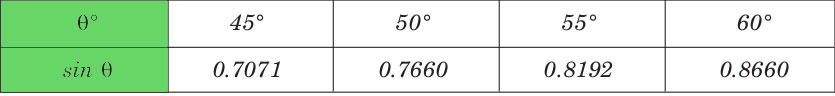Output :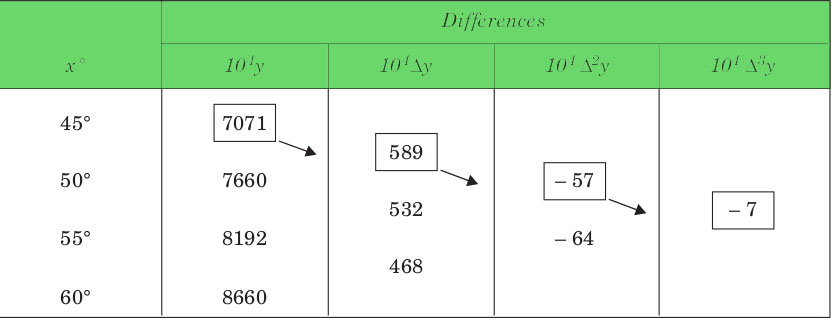Value at Sin 52 is 0.788003


Below is the implementation of newton forward interpolation method.

## C++

 // CPP Program to interpolate using   // newton forward interpolation  #include  using namespace std;     // calculating u mentioned in the formula  float u_cal(float u, int n)  {      float temp = u;      for (int i = 1; i < n; i++)          temp = temp * (u - i);      return temp;  }     // calculating factorial of given number n  int fact(int n)  {      int f = 1;      for (int i = 2; i <= n; i++)          f *= i;      return f;  }     int main()  {      // Number of values given      int n = 4;      float x[] = { 45, 50, 55, 60 };             // y[][] is used for difference table      // with y[] used for input      float y[n][n];      y = 0.7071;      y = 0.7660;      y = 0.8192;      y = 0.8660;         // Calculating the forward difference      // table      for (int i = 1; i < n; i++) {          for (int j = 0; j < n - i; j++)              y[j][i] = y[j + 1][i - 1] - y[j][i - 1];      }         // Displaying the forward difference table      for (int i = 0; i < n; i++) {          cout << setw(4) << x[i]                << "\t";          for (int j = 0; j < n - i; j++)              cout << setw(4) << y[i][j]                    << "\t";          cout << endl;      }         // Value to interpolate at      float value = 52;         // initializing u and sum      float sum = y;      float u = (value - x) / (x - x);      for (int i = 1; i < n; i++) {          sum = sum + (u_cal(u, i) * y[i]) /                                   fact(i);      }         cout << "\n Value at " << value << " is "           << sum << endl;      return 0;  }

## Java

 // Java Program to interpolate using   // newton forward interpolation     class GFG{  // calculating u mentioned in the formula  static double u_cal(double u, int n)  {      double temp = u;      for (int i = 1; i < n; i++)          temp = temp * (u - i);      return temp;  }     // calculating factorial of given number n  static int fact(int n)  {      int f = 1;      for (int i = 2; i <= n; i++)          f *= i;      return f;  }     public static void main(String[] args)  {      // Number of values given      int n = 4;      double x[] = { 45, 50, 55, 60 };             // y[][] is used for difference table      // with y[] used for input      double y[][]=new double[n][n];      y = 0.7071;      y = 0.7660;      y = 0.8192;      y = 0.8660;         // Calculating the forward difference      // table      for (int i = 1; i < n; i++) {          for (int j = 0; j < n - i; j++)              y[j][i] = y[j + 1][i - 1] - y[j][i - 1];      }         // Displaying the forward difference table      for (int i = 0; i < n; i++) {          System.out.print(x[i]+"\t");          for (int j = 0; j < n - i; j++)              System.out.print(y[i][j]+"\t");          System.out.println();      }         // Value to interpolate at      double value = 52;         // initializing u and sum      double sum = y;      double u = (value - x) / (x - x);      for (int i = 1; i < n; i++) {          sum = sum + (u_cal(u, i) * y[i]) /                                  fact(i);      }         System.out.println("\n Value at "+value+" is "+String.format("%.6g%n",sum));  }  }  // This code is contributed by mits

## Python3

 # Python3 Program to interpolate using   # newton forward interpolation     # calculating u mentioned in the formula  def u_cal(u, n):         temp = u;      for i in range(1, n):          temp = temp * (u - i);      return temp;     # calculating factorial of given number n  def fact(n):      f = 1;      for i in range(2, n + 1):          f *= i;      return f;     # Driver Code     # Number of values given  n = 4;  x = [ 45, 50, 55, 60 ];         # y[][] is used for difference table  # with y[] used for input  y = [[0 for i in range(n)]          for j in range(n)];  y = 0.7071;  y = 0.7660;  y = 0.8192;  y = 0.8660;     # Calculating the forward difference  # table  for i in range(1, n):      for j in range(n - i):          y[j][i] = y[j + 1][i - 1] - y[j][i - 1];     # Displaying the forward difference table  for i in range(n):      print(x[i], end = "\t");      for j in range(n - i):          print(y[i][j], end = "\t");      print("");     # Value to interpolate at  value = 52;     # initializing u and sum  sum = y;  u = (value - x) / (x - x);  for i in range(1,n):      sum = sum + (u_cal(u, i) * y[i]) / fact(i);     print("\nValue at", value,         "is", round(sum, 6));     # This code is contributed by mits

## C#

 // C# Program to interpolate using   // newton forward interpolation  using System;     class GFG  {  // calculating u mentioned in the formula  static double u_cal(double u, int n)  {      double temp = u;      for (int i = 1; i < n; i++)          temp = temp * (u - i);      return temp;  }     // calculating factorial of given number n  static int fact(int n)  {      int f = 1;      for (int i = 2; i <= n; i++)          f *= i;      return f;  }     // Driver code  public static void Main()  {      // Number of values given      int n = 4;      double[] x = { 45, 50, 55, 60 };             // y[,] is used for difference table      // with y[,0] used for input      double[,] y=new double[n,n];      y[0,0] = 0.7071;      y[1,0] = 0.7660;      y[2,0] = 0.8192;      y[3,0] = 0.8660;         // Calculating the forward difference      // table      for (int i = 1; i < n; i++) {          for (int j = 0; j < n - i; j++)              y[j,i] = y[j + 1,i - 1] - y[j,i - 1];      }         // Displaying the forward difference table      for (int i = 0; i < n; i++) {          Console.Write(x[i]+"\t");          for (int j = 0; j < n - i; j++)              Console.Write(y[i,j]+"\t");          Console.WriteLine();      }         // Value to interpolate at      double value = 52;         // initializing u and sum      double sum = y[0,0];      double u = (value - x) / (x - x);      for (int i = 1; i < n; i++) {          sum = sum + (u_cal(u, i) * y[0,i]) /                                  fact(i);      }         Console.WriteLine("\n Value at "+value+" is "+Math.Round(sum,6));  }  }  // This code is contributed by mits

## PHP

 

Output:

  45    0.7071    0.0589    -0.00569999    -0.000699997
50    0.766    0.0532    -0.00639999
55    0.8192    0.0468
60    0.866

Value at 52 is 0.788003


Backward Differences : The differences y1 – y0, y2 – y1, ……, yn – yn–1 when denoted by dy1, dy2, ……, dyn, respectively, are called first backward difference. Thus the first backward differences are :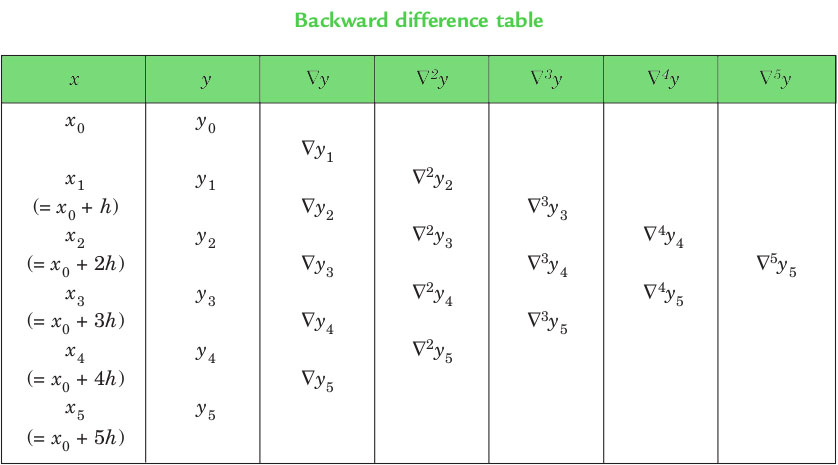NEWTON’S GREGORY BACKWARD INTERPOLATION FORMULA :This formula is useful when the value of f(x) is required near the end of the table. h is called the interval of difference and u = ( x – an ) / h, Here an is last term.

Example :

Input : Population in 1925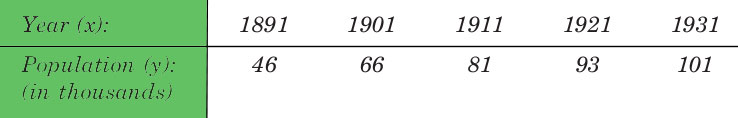Output :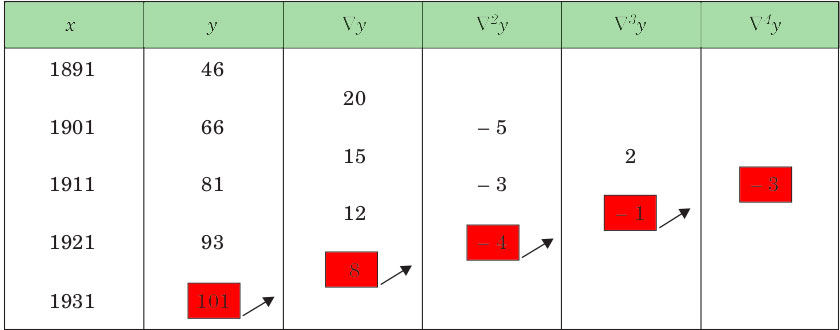Value in 1925 is 96.8368


Below is the implementation of newton backward interpolation method.

## C++

 // CPP Program to interpolate using  // newton backward interpolation  #include  using namespace std;     // Calculation of u mentioned in formula  float u_cal(float u, int n)  {      float temp = u;      for (int i = 1; i < n; i++)          temp = temp * (u + i);      return temp;  }     // Calculating factorial of given n  int fact(int n)  {      int f = 1;      for (int i = 2; i <= n; i++)          f *= i;      return f;  }     int main()  {      // number of values given      int n = 5;      float x[] = { 1891, 1901, 1911,                     1921, 1931 };                           // y[][] is used for difference       // table and y[] used for input      float y[n][n];      y = 46;      y = 66;      y = 81;      y = 93;      y = 101;         // Calculating the backward difference table      for (int i = 1; i < n; i++) {          for (int j = n - 1; j >= i; j--)              y[j][i] = y[j][i - 1] - y[j - 1][i - 1];      }         // Displaying the backward difference table      for (int i = 0; i < n; i++) {          for (int j = 0; j <= i; j++)              cout << setw(4) << y[i][j]                    << "\t";          cout << endl;      }         // Value to interpolate at      float value = 1925;         // Initializing u and sum      float sum = y[n - 1];      float u = (value - x[n - 1]) / (x - x);      for (int i = 1; i < n; i++) {          sum = sum + (u_cal(u, i) * y[n - 1][i]) /                                       fact(i);      }         cout << "\n Value at " << value << " is "           << sum << endl;      return 0;  } 

## Java

 // Java Program to interpolate using  // newton backward interpolation  class GFG  {         // Calculation of u mentioned in formula  static double u_cal(double u, int n)  {      double temp = u;      for (int i = 1; i < n; i++)          temp = temp * (u + i);      return temp;  }     // Calculating factorial of given n  static int fact(int n)  {      int f = 1;      for (int i = 2; i <= n; i++)          f *= i;      return f;  }     // Driver code  public static void main(String[] args)  {      // number of values given      int n = 5;      double x[] = { 1891, 1901, 1911,                   1921, 1931 };                         // y[][] is used for difference       // table and y[] used for input      double[][] y = new double[n][n];      y = 46;      y = 66;      y = 81;      y = 93;      y = 101;         // Calculating the backward difference table      for (int i = 1; i < n; i++)       {          for (int j = n - 1; j >= i; j--)              y[j][i] = y[j][i - 1] - y[j - 1][i - 1];      }         // Displaying the backward difference table      for (int i = 0; i < n; i++)       {          for (int j = 0; j <= i; j++)              System.out.print(y[i][j] + "\t");          System.out.println("");;      }         // Value to interpolate at      double value = 1925;         // Initializing u and sum      double sum = y[n - 1];      double u = (value - x[n - 1]) / (x - x);      for (int i = 1; i < n; i++)       {          sum = sum + (u_cal(u, i) * y[n - 1][i]) /                                      fact(i);      }      System.out.println("\n Value at " + value +                       " is " + String.format("%.6g%n",sum));  }  }     // This code is contributed by mits 

## C#

 // C# Program to interpolate using  // newton backward interpolation  using System;     class GFG  {         // Calculation of u mentioned in formula  static double u_cal(double u, int n)  {      double temp = u;      for (int i = 1; i < n; i++)          temp = temp * (u + i);      return temp;  }     // Calculating factorial of given n  static int fact(int n)  {      int f = 1;      for (int i = 2; i <= n; i++)          f *= i;      return f;  }     // Driver code  static void Main()  {      // number of values given      int n = 5;      double[] x = { 1891, 1901, 1911,                   1921, 1931 };                         // y[][] is used for difference       // table and y[] used for input      double[,] y = new double[n,n];      y[0,0] = 46;      y[1,0] = 66;      y[2,0] = 81;      y[3,0] = 93;      y[4,0] = 101;         // Calculating the backward difference table      for (int i = 1; i < n; i++)       {          for (int j = n - 1; j >= i; j--)              y[j,i] = y[j,i - 1] - y[j - 1,i - 1];      }         // Displaying the backward difference table      for (int i = 0; i < n; i++)      {          for (int j = 0; j <= i; j++)              Console.Write(y[i,j]+"\t");          Console.WriteLine("");;      }         // Value to interpolate at      double value = 1925;         // Initializing u and sum      double sum = y[n - 1,0];      double u = (value - x[n - 1]) / (x - x);      for (int i = 1; i < n; i++)       {          sum = sum + (u_cal(u, i) * y[n - 1,i]) /                                      fact(i);      }         Console.WriteLine("\n Value at "+value+" is "+Math.Round(sum,4));  }  }     // This code is contributed by mits 

## PHP

 = $i; $j--)          $y[$j][$i] = $y[$j][$i - 1] -                       $y[$j - 1][$i - 1];  }    // Displaying the backward difference table  for ($i = 0; $i < $n; $i++)  {   for ($j = 0; $j <= $i; $j++)   print($y[$i][$j] . "\t");      print("\n");  }     // Value to interpolate at  $value = 1925;    // Initializing u and sum  $sum = $y[$n - 1];  $u = ($value - $x[$n - 1]) / ($x - $x);  for ($i = 1; $i < $n; $i++)   {      $sum = $sum + (u_cal($u, $i) *              $y[$n - 1][$i]) / fact($i);  }     print("\n Value at " . $value .   " is " . round($sum, 4));     // This code is contributed by chandan_jnu  ?> 

Output:

  46
66      20
81      15      -5
93      12      -3       2
101       8      -4      -1      -3

Value at 1925 is 96.8368
`

This article is contributed by Shubham Rana. If you like GeeksforGeeks and would like to contribute, you can also write an article using contribute.geeksforgeeks.org or mail your article to contribute@geeksforgeeks.org. See your article appearing on the GeeksforGeeks main page and help other Geeks.

Attention reader! Don’t stop learning now. Get hold of all the important DSA concepts with the DSA Self Paced Course at a student-friendly price and become industry ready.

My Personal Notes arrow_drop_up

Article Tags :
Practice Tags :

3

Please write to us at contribute@geeksforgeeks.org to report any issue with the above content.